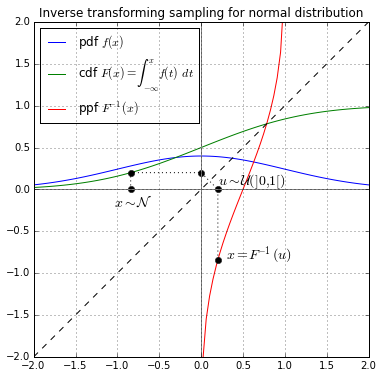# Python Decorators with Data Science: Random SamplingThis post is more educational in terms of learning a few concepts together. I was wondering how can I use python decorators with a specific Data Science concept and the idea for this post was born. In this post, I won’t go into that but just use the inverse transform to compute random samples for a few distributions.

This post is more educational in terms of learning a few concepts together. I was wondering how can I use python decorators with a specific Data Science concept and the idea for this post was born. Let us first look into the random sampling on how it is done.

## Random Sampling

The inverse transform is one of the methods to generate random samples from some of the well-known distributions. Inverse transformation takes uniform samples u between 0 and 1 and returns the largest number x from distribution P(X) such that the probability of X below _x _is less than equal to u.

The [probability integral transform_](https://en.wikipedia.org/wiki/Probability_integral_transform) states that if X is a [continuous random variable_](https://en.wikipedia.org/wiki/Continuous_random_variable)_ with [cumulative distribution function_](https://en.wikipedia.org/wiki/Cumulative_distribution_function)_ Fₓ , then the random variable Y=Fₓ(X) has a [uniform distribution_](https://en.wikipedia.org/wiki/Uniform_distribution_(continuous))_ on [0, 1]. The inverse probability integral transform is just the inverse of this: specifically, if Y has a uniform distribution on [0, 1] and if X has a cumulative distribution Fₓ , then the random variable Fₓ⁻¹(Y) has the same distribution as X._

## Data Science Projects | Data Science | Machine Learning | Python

Practice your skills in Data Science with Python, by learning and then trying all these hands-on, interactive projects, that I have posted for you.

## Data Science Projects | Data Science | Machine Learning | Python

Practice your skills in Data Science with Python, by learning and then trying all these hands-on, interactive projects, that I have posted for you.

## Data Science Projects | Data Science | Machine Learning | Python

Practice your skills in Data Science with Python, by learning and then trying all these hands-on, interactive projects, that I have posted for you.

## Data Science Projects | Data Science | Machine Learning | Python

Practice your skills in Data Science with Python, by learning and then trying all these hands-on, interactive projects, that I have posted for you.

## Data Science Projects | Data Science | Machine Learning | Python

Practice your skills in Data Science with Python, by learning and then trying all these hands-on, interactive projects, that I have posted for you.